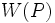# Weakly closed conjugacy functor

This article defines a property that can be evaluated for a conjugacy functor on a finite group. |View all such properties

## Definition

Suppose$G$ is a finite group,$p$ a prime number, and$W$ a conjugacy functor on$G$ with respect to$p$. We say that$W$ is weakly closed in$G$ with respect to$p$ if the following equivalent conditions are satisfied:

1. Either of these equivalent:
• There exists a$p$-Sylow subgroup$P$ of$G$ such that$W(P)$ is a weakly closed subgroup of$P$ relative to$G$.
• For every$p$-Sylow subgroup$P$ of$G$,$W(P)$ is a weakly closed subgroup of$P$ relative to$G$.
2. Either of these equivalent:
• There exists a$p$-Sylow subgroup$P$ such that, for every$p$-Sylow subgroup$Q$ containing$W(P)$,$W(P) = W(Q)$.
• For every$p$-Sylow subgroup$P$, and for every$p$-Sylow subgroup$Q$ containing$W(P)$,$W(P) = W(Q)$.
3. Either of these equivalent:
• There exists a$p$-Sylow subgroup$P$ of$G$ such that for any$p$-Sylow subgroup$Q$ of$G$ containing$W(P)$,$W(P)$ is a normal subgroup of$Q$.
• For every$p$-Sylow subgroup$P$ of$G$, it is true that for any$p$-Sylow subgroup$Q$ of$G$ containing$W(P)$,$W(P)$ is a normal subgroup of$Q$.

For instance, a p-normal group is a group in which the conjugacy functor that arises by taking the center is weakly closed.

### Equivalence of definitions

Further information: equivalence of definitions of weakly closed conjugacy functor